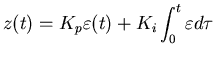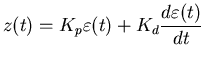Next: The PID controller Up: number7 Previous: The Proportional Controller

# Improving the Controller

We can reduce the tendency of the control signal to oscilate about the desired position by giving the controller some memory. We accomplish this by adding a term that keeps track of the accumulated error over time,The integral in this equation provides the memory. This type of controller is known as the proportional-integral or P-I controller.

Since the integral accumulates the error from the beginning there is the possibility of an overflow in this term, especially if an integer only implementation is used and/or the sample rate is high. For integer implementations there are two common tricks that are used to reduce the overflow problem: (i) whenever an overflow is detected reduce the accumulated sum by half and increase the gain by two, and (ii) put limits on the integral term and just stop accumulating when these bounds are reached.

Another improvement that we can add the basic proportional controller is to make it more responsive to variations in the control signal. We can do this by adding a term that is large when the control changes, i.e. we add a derivative term,If we just add the derivative as above, we get the proportional-derivative, or P-D controller.Next: The PID controller Up: number7 Previous: The Proportional Controller
Skip Carter 2008-08-20